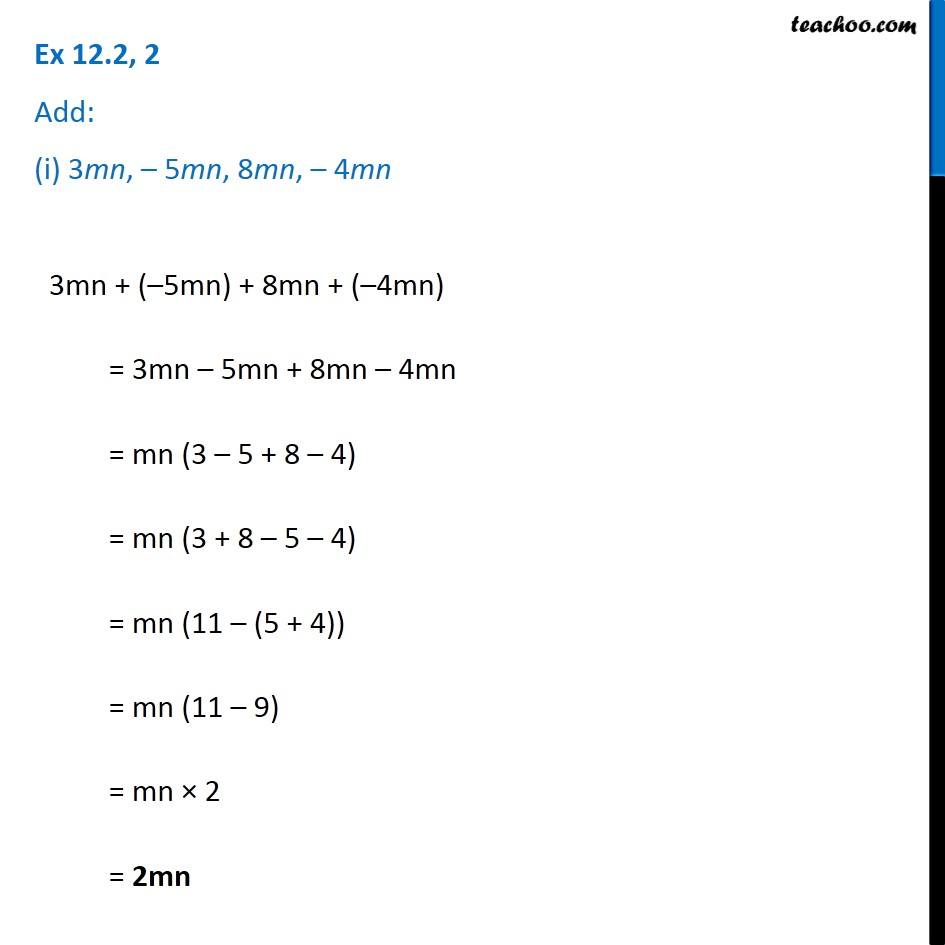Addition and Subtraction of Algebraic Expressions

Chapter 10 Class 7 Algebraic Expressions
Concept wiseGet live Maths 1-on-1 Classs - Class 6 to 12

### Transcript

Question 2 Add: (i) 3mn, – 5mn, 8mn, – 4mn 3mn + (–5mn) + 8mn + (–4mn) = 3mn – 5mn + 8mn – 4mn = mn (3 – 5 + 8 – 4) = mn (3 + 8 – 5 – 4) = mn (11 – (5 + 4)) = mn (11 – 9) = mn × 2 = 2mn 1